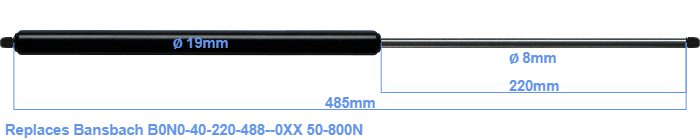# Replacement for Bansbach B0N0-40-220-488–0XX 50-800N

\$45.80

Replacement gas spring for the Bansbach B0N0-40-220-488--0XX 50-800 Newton. Thread: M8. Brand: Stabilus Industry Line.
 Force Choose an option50 Newton60 Newton80 Newton100 Newton120 Newton140 Newton150 Newton160 Newton180 Newton200 Newton220 Newton240 Newton250 Newton260 Newton280 Newton300 Newton320 Newton340 Newton350 Newton360 Newton380 Newton400 Newton420 Newton440 Newton450 Newton460 Newton480 Newton500 Newton520 Newton540 Newton550 Newton560 Newton580 Newton600 Newton620 Newton640 Newton650 Newton660 Newton680 Newton700 Newton720 Newton740 Newton750 Newton760 Newton780 Newton800 NewtonClearThe diameter of the cylinder of this gas spring is 19 mm. The diameter of the rod is 8 millimeter. The chrome part is called the rod. It has a length of 220 mm. The total length of this gas strut is 485 mm. This is the total distance between the centers of the two mounting parts. The distance from thread to thread (without mounting parts) is 485 mm. The force of this replacement gas spring is 50-800 Newton. This gas spring can replace a Bansbach gas spring, but is not an orignal Bansbach one. HAHN Gasfedern, a Stabilus subsidiary, is responsible for the production of this replacement gas spring. The gas spring has the brand Stabilus Industry Line. Nevertheless, the dimensions and force are equal.